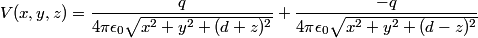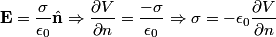## Solution to 1986 Problem 63

 The potential above the plane is easily found by placing an image charge below the plane.\begin{align*}V(x,y,z) = \frac{q}{4 \pi \epsilon_0\sqrt{x^2 + y^2 + (d + z)^2}} + \frac{-q}{4 \pi \epsilon_0\sqrt{x^2 + y^2 +...The induced charge density on the conducting plane can be found using Gauss's Law\begin{align*}\mathbf{E} = \frac{\sigma}{\epsilon_0}\hat{\mathbf{n}} \Rightarrow \frac{\partial V}{\partial n} = \frac{-\sigm...Therefore,\begin{align*}\sigma = - \epsilon_0 \frac{\partial V}{\partial n} = \boxed{\frac{q d}{2 \pi D^3}} \end{align*}Therefore, answer (D) is correct.